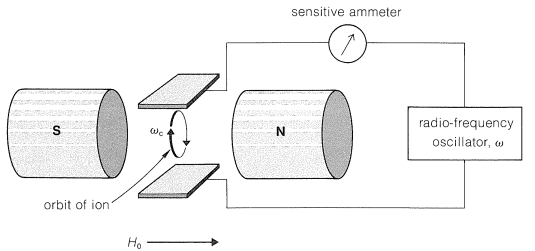27.9: Ion-Cyclotron Resonance

A gaseous ion in a magnetic field moves in a circular orbit with an angular frequency $$\omega_c$$ such that $$\omega_c = \left( e/m \right) \left( H_0/c \right)$$, in which $$e/m$$ is the ratio of charge to mass, $$H_0$$ is the applied magnetic field, and $$c$$ is the velocity of light. The frequency $$\omega_c$$ is called the "cyclotron frequency" and is the basis of the cyclotron particle accelerator used in nuclear physics. Now suppose a radio-frequency field is imposed on the ions from a variable oscillator, as shown in Figure 27-15. When the frequency of the oscillator $$\omega$$ equals $$\omega_c$$, the ions absorb energy and move faster through larger orbits, but at the same frequency $$\omega_c$$.Figure 27-15: Detection of ion-cyclotron resonance. When $$\omega = \omega_c$$, energy is absorbed by the ions and the ammeter registers a current.

Ion-cyclotron resonance combines features of mass spectroscopy in that the ratio $$e/m$$ is involved, and of NMR spectroscopy in that detection depends on absorption of energy from a radio-frequency oscillator. The chemical applications depend on reactions between the ions during the time they remain in the cyclotron, which may be many seconds. Suppose then that we generate $$\ce{OH}^\ominus$$ by electron bombardment of a gaseous mixture of water and 2-methyl-2-propanol (tert-butyl alcohol). The $$\ce{OH}^\ominus$$ ion can be detected by its characteristic frequency $$\omega = \left( e/m \right) \left( H_0/c \right)$$, in which $$e/m = 1/17$$. Now, because the reaction $$\ce{(CH_3)_3COH} + \ce{OH}^\ominus \rightarrow \ce{(CH_3)_3CO}^\ominus + \ce{H_2O}$$ occurs, a new ion of $$e/m = 1/73$$ appears. The reverse reaction, $$\ce{(CH_3)_3CO}^\ominus + \ce{H_2O} \rightarrow \ce{(CH_3)_3COH} + \ce{OH}^\ominus$$, does not occur to a measurable extent. From this we can infer that $$\ce{(CH_3)_3COH}$$ is a stronger acid than $$\ce{H_2O}$$ in the gas phase. These experiments clearly are related to chemical-ionization mass spectroscopy (Section 27-7), and provide the basis for determining the gas-phase acidities of alkynes and water, discussed in Section 11-8. A detailed gas-phase acidity scale has been established by this means.

Many unusual reactions occur between ions and neutral molecules in the gas phase, which can be detected by ion-cyclotron resonance; a few examples are

$\ce{CH_3F^+} \: \text{(from electron impact)} + \ce{CH_3F} \rightarrow \ce{CH_3} \overset{+}{\ce{F}} \ce{H} + \cdot \ce{CH_2F} \: \text{(} \ce{H} \cdot \: \text{atom transfer)}$

$\ce{CH_3FH^+} + \ce{N_2} \rightarrow \ce{CH_3N_2^+} + \ce{HF} \: \: \: \: \: \text{(nucleophilic displacement)}$

$\ce{CH_3FH^+} + \ce{Xe} \rightarrow \ce{CH_3Xe^+} + \ce{HF} \: \: \: \: \: \text{(nucleophilic displacement)}$

Clearly, in gas-phase reactions $$\ce{HF}$$ is an extremely good leaving group in being rapidly displaced both by $$\ce{Xe}$$ and $$\ce{N_2}$$. From our discussions of leaving groups in Section 8-7C, we can infer that $$\ce{H_2F}^\oplus$$ must be a very strong acid in the gas phase and the available evidence indicates that this is so.

It is possible to measure the concentrations of the ions as a function of time and thus determine the rates of reaction of ions with neutral molecules in the gas phase. Figure 27-16 shows the results of a typical experiment wherein a sequence of reactions occurs that involves chloromethane as the neutral molecule and begins with the ion $$\ce{CH_3Cl}^\oplus$$ formed by a short burst $$\left( 10 \: \text{msec} \right)$$ of $$16 \: \text{KeV}$$ electrons. The originally formed $$\ce{CH_3Cl}^\oplus$$ ions react with $$\ce{CH_3Cl}$$ to yield $$\ce{CH_3ClH}^\oplus + \cdot \ce{CH_2Cl}$$. The buildup of $$\ce{CH_3ClH}^\oplus$$ and the disappearance of $$\ce{CH_3Cl}^\oplus$$ clearly are coupled. A slower reaction, $$\ce{CH_3ClH}^\oplus + \ce{CH_3Cl} \rightarrow \ce{(CH_3)_2Cl}^\oplus + \ce{HCl}$$, then takes over the action.